# Batch Thickening Theory

Kynch showed that a constant concentration layer propogates upward at constant rate in batch thickening tests under the assumption that settling velocity is a function of only the concentration, as described earlier under Continuous Thickening Theory. His conclusion can be expressed as: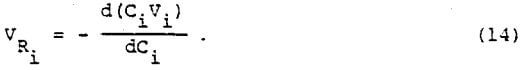Where Ci is the concentration of solids in the layer, and Vi is the settling velocity of the layer. VRi is the rate of upward propogation of the constant concentration layer.

The following equations are obtained by material balance: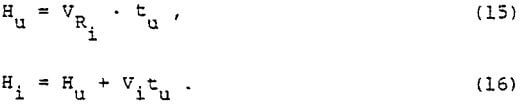Where tu is the settling time, Hu is the interface height at tu, and Hi is the intercept of the tangent to the settling curve with the interface height axis. The relationship of the above equations is illustrated in Figure 6.

The average concentration, Cu, of the slurry bed at the settling time tu is expressed as: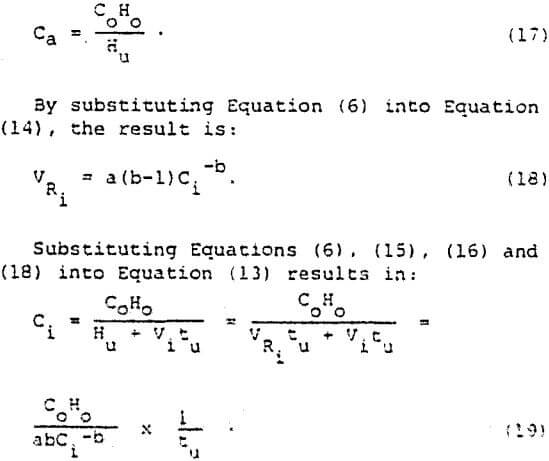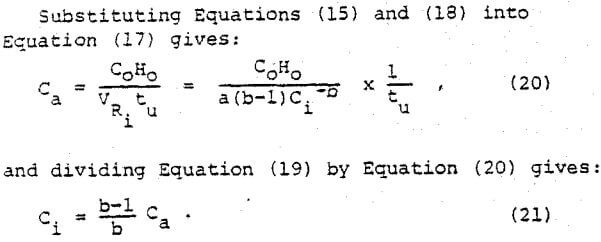Note that Equation (21) for batch thickening has the same form as Equation (10) for continuous thickening where CL corresponds to Ci and Cu corresponds to Ca.

Talmage and Fitch obtained the unit area from batch settling curves using the following equation:

(U.A.)B = tx/CoHo……………………………..(22)

They defined the “underflow line” as a horizontal line calculated from the underflow concentration desired in the continuous thickener as:

Hu = CoHo/Cu……………………………….(23)

By comparing Equation (23) with Equation (17) , it should be noted that the underflow concentration desired, Cu, is equal to the average concentration, Ca, at the intercept of the “underflow line” with the settling curve. In order to determine the special settling time, tx, in Equation (22), Talmage and Fitch suggested that tx is the intercept of the tangent to the batch curve at the “compression point” with the “underflow line.”

The special point, tx, is now assumed to be equal to tu in order to obtain the real meaning of tx. Substituting Equations (15), (17) and (18) into Equation (22) evaluated where tx = tu, results in: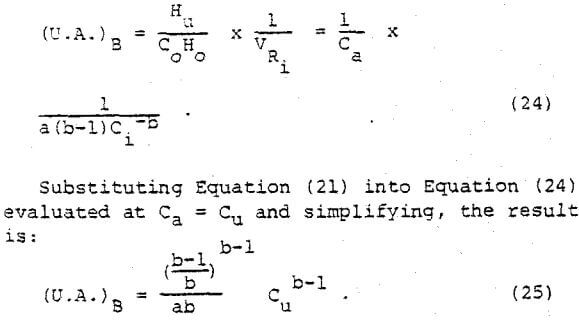A comparison of Equation (25) and Equation (12) brings out some important relationships. The two equations give identical unit areas. This verifies the assumption that tx = tu used previously. It is first concluded that the intersection in the time axis of the underflow line with the settling curve must be used as tx in Equation (22) to predict the full-scale unit area. Any other value of tx taken from tangents to the batch curve such as the Talmage and Fitch method would not accurately predict the continuous thickener performance as described by Equation (12). Secondly, the underflow concentration in the continuous thickener corresponds to the average concentration of the slurry bed in the batch tests, and the limiting concentration in the continuous thickener corresponds to the concentration just below the interface in the batch tests.